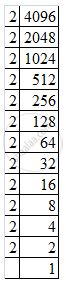Advertisement Remove all ads

# Find the Square Root the Following by Prime Factorization. 4096 - Mathematics

Find the square root  the following by prime factorization.

4096

Advertisement Remove all ads

#### Solution

Resolving 4096 into prime factors:
4096 = 2 x 2 x 2 x 2 x 2 x 2 x 2 x 2 x 2 x 2 x 2 x 2Grouping the factors into pairs of equal factors:
4096 = (2 x 2) x (2 x 2) x (2 x 2) x (2 x 2) x (2 x 2) x (2 x 2)
Taking one factor for each pair, we get the square root of 4096:
(2 x 2) x (2 x 2) x (2 x 2) = 64

Is there an error in this question or solution?
Advertisement Remove all ads

#### APPEARS IN

RD Sharma Class 8 Maths
Chapter 3 Squares and Square Roots
Exercise 3.4 | Q 2.06 | Page 38
Advertisement Remove all ads

#### Video TutorialsVIEW ALL 

Advertisement Remove all ads
Share
Notifications

View all notifications

Forgot password?
Course